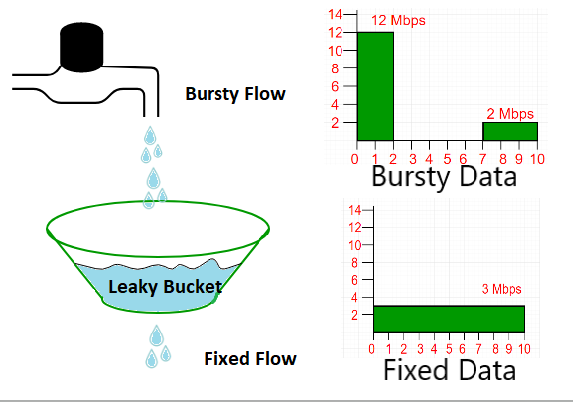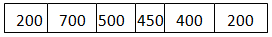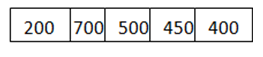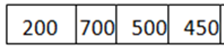Open in App
Not now

## Related Articles

• Write an Interview Experience

# Computer Network | Leaky bucket algorithm

• Difficulty Level : Easy
• Last Updated : 31 Jan, 2023

In the network layer, before the network can make Quality of service guarantees, it must know what traffic is being guaranteed. One of the main causes of congestion is that traffic is often bursty.

To understand this concept first we have to know little about traffic shaping. Traffic Shaping is a mechanism to control the amount and the rate of traffic sent to the network. Approach of congestion management is called Traffic shaping. Traffic shaping helps to regulate the rate of data transmission and reduces congestion.

There are 2 types of traffic shaping algorithms:

1. Leaky Bucket
2. Token Bucket

Suppose we have a bucket in which we are pouring water, at random points in time, but we have to get water at a fixed rate, to achieve this we will make a hole at the bottom of the bucket. This will ensure that the water coming out is at some fixed rate, and also if the bucket gets full, then we will stop pouring water into it.

The input rate can vary, but the output rate remains constant. Similarly, in networking, a technique called leaky bucket can smooth out bursty traffic. Bursty chunks are stored in the bucket and sent out at an average rate.In the above figure, we assume that the network has committed a bandwidth of 3 Mbps for a host. The use of the leaky bucket shapes the input traffic to make it conform to this commitment. In the above figure, the host sends a burst of data at a rate of 12 Mbps for 2s, for a total of 24 Mbits of data. The host is silent for 5 s and then sends data at a rate of 2 Mbps for 3 s, for a total of 6 Mbits of data. In all, the host has sent 30 Mbits of data in 10 s. The leaky bucket smooths out the traffic by sending out data at a rate of 3 Mbps during the same 10 s.

Without the leaky bucket, the beginning burst may have hurt the network by consuming more bandwidth than is set aside for this host. We can also see that the leaky bucket may prevent congestion.

A simple leaky bucket algorithm can be implemented using FIFO queue. A FIFO queue holds the packets. If the traffic consists of fixed-size packets (e.g., cells in ATM networks), the process removes a fixed number of packets from the queue at each tick of the clock. If the traffic consists of variable-length packets, the fixed output rate must be based on the number of bytes or bits.

The following is an algorithm for variable-length packets:

1. Initialize a counter to n at the tick of the clock.
2. Repeat until n is smaller than the packet size of the packet at the head of the queue.
1. Pop a packet out of the head of the queue, say P.
2. Send the packet P, into the network
3. Decrement the counter by the size of packet P.
3. Reset the counter and go to step 1.

Note: In the below examples, the head of the queue is the rightmost position and the tail of the queue is the leftmost position.

Example: Let n=1000

Packet=Since n > size of the packet at the head of the Queue, i.e. n > 200
Therefore, n = 1000-200 = 800
Packet size of 200 is sent into the network.Now, again n > size of the packet at the head of the Queue, i.e. n > 400
Therefore, n = 800-400 = 400
Packet size of 400 is sent into the network.Since, n < size of the packet at the head of the Queue, i.e.  n < 450
Therefore, the procedure is stopped.

Initialise n = 1000 on another tick of the clock.
This procedure is repeated until all the packets are sent into the network.

Below is the implementation of above explained approach:

## C++

 `// cpp program to implement leakybucket``#include ``using` `namespace` `std;``int` `main()``{``    ``int` `no_of_queries, storage, output_pkt_size;``    ``int` `input_pkt_size, bucket_size, size_left;` `    ``// initial packets in the bucket``    ``storage = 0;` `    ``// total no. of times bucket content is checked``    ``no_of_queries = 4;` `    ``// total no. of packets that can``    ``// be accommodated in the bucket``    ``bucket_size = 10;` `    ``// no. of packets that enters the bucket at a time``    ``input_pkt_size = 4;` `    ``// no. of packets that exits the bucket at a time``    ``output_pkt_size = 1;``    ``for` `(``int` `i = 0; i < no_of_queries; i++) ``// space left``    ``{``        ``size_left = bucket_size - storage;``        ``if` `(input_pkt_size <= size_left) {``            ``// update storage``            ``storage += input_pkt_size;``        ``}``        ``else` `{``            ``printf``(``"Packet loss = %d\n"``, input_pkt_size);``        ``}``        ``printf``(``"Buffer size= %d out of bucket size= %d\n"``,``               ``storage, bucket_size);``        ``storage -= output_pkt_size;``    ``}``    ``return` `0;``}` `// This code is contributed by bunny09262002``// Improved by: rishitchaudhary`

## Java

 `// Java Implementation of Leaky bucket` `import` `java.io.*;``import` `java.util.*;` `class` `Leakybucket {``    ``public` `static` `void` `main(String[] args)``    ``{``        ``int` `no_of_queries, storage, output_pkt_size;``        ``int` `input_pkt_size, bucket_size, size_left;` `        ``// initial packets in the bucket``        ``storage = ``0``;` `        ``// total no. of times bucket content is checked``        ``no_of_queries = ``4``;` `        ``// total no. of packets that can``        ``// be accommodated in the bucket``        ``bucket_size = ``10``;` `        ``// no. of packets that enters the bucket at a time``        ``input_pkt_size = ``4``;` `        ``// no. of packets that exits the bucket at a time``        ``output_pkt_size = ``1``;``        ``for` `(``int` `i = ``0``; i < no_of_queries; i++) {``            ``size_left = bucket_size - storage; ``// space left` `            ``if` `(input_pkt_size <= (size_left)) {``                ``storage += input_pkt_size;``            ``}``            ``else` `{``                ``System.out.println(``"Packet loss = "``                                   ``+ input_pkt_size);``            ``}``            ``System.out.println(``"Buffer size= "` `+ storage``                               ``+ ``" out of bucket size= "``                               ``+ bucket_size);``            ``storage -= output_pkt_size;``        ``}``    ``}``}``// Improved by: rishitchaudhary`

## Python3

 `# initial packets in the bucket``storage ``=` `0` `# total no. of times bucket content is checked``no_of_queries ``=` `4` `# total no. of packets that can``# be accommodated in the bucket``bucket_size ``=` `10` `# no. of packets that enters the bucket at a time``input_pkt_size ``=` `4` `# no. of packets that exits the bucket at a time``output_pkt_size ``=` `1``for` `i ``in` `range``(``0``, no_of_queries):  ``# space left` `    ``size_left ``=` `bucket_size ``-` `storage``    ``if` `input_pkt_size <``=` `size_left:``      ``# update storage``        ``storage ``+``=` `input_pkt_size``    ``else``:``        ``print``(``"Packet loss = "``, input_pkt_size)` `    ``print``(f``"Buffer size= {storage} out of bucket size = {bucket_size}"``)` `    ``# as packets are sent out into the network, the size of the storage decreases``    ``storage ``-``=` `output_pkt_size`  `# This code is contributed by Arpit Jain``# Improved by: rishitchaudhary`

## C#

 `// C# Implementation of Leaking bucket``using` `System;` `class` `Leakingbucket``{``  ``static` `void` `Main(``string``[] args)``  ``{``    ``int` `no_of_queries, storage, output_pkt_size;``    ``int` `input_pkt_size, bucket_size, size_left;` `    ``// initial packets in the bucket``    ``storage = 0;` `    ``// total no. of times bucket content is checked``    ``no_of_queries = 4;` `    ``// total no. of packets that can``    ``// be accommodated in the bucket``    ``bucket_size = 10;` `    ``// no. of packets that enters the bucket at a time``    ``input_pkt_size = 4;` `    ``// no. of packets that exits the bucket at a time``    ``output_pkt_size = 1;``    ``for` `(``int` `i = 0; i < no_of_queries; i++)``    ``{``      ``size_left = bucket_size - storage; ``// space left` `      ``if` `(input_pkt_size <= (size_left))``      ``{``        ``storage += input_pkt_size;``      ``}``      ``else``      ``{``        ``Console.WriteLine(``"Packet loss = "` `+ input_pkt_size);``      ``}``      ``Console.WriteLine(``"Buffer size= "` `+ storage + ``" out of bucket size= "` `+ bucket_size);``      ``storage -= output_pkt_size;``    ``}``  ``}``}` `// This code is Contributed by phasing17`

## Javascript

 `// JS code to implement the approach` `// initial packets in the bucket``let storage = 0;` `// total no. of times bucket content is checked``let noOfQueries = 4;` `// total no. of packets that can``// be accommodated in the bucket``let bucketSize = 10;` `// no. of packets that enters the bucket at a time``let inputPktSize = 4;` `// no. of packets that exits the bucket at a time``let outputPktSize = 1;` `for` `(let i = 0; i < noOfQueries; i++) { ``// space left` `let sizeLeft = bucketSize - storage;``if` `(inputPktSize <= sizeLeft) {``// update storage``storage += inputPktSize;``} ``else` `{``console.log(``"Packet loss = "``, inputPktSize);``}` `console.log(`Buffer size= \${storage} out of bucket size = \${bucketSize}`);` `// as packets are sent out into the network, the size of the storage decreases``storage -= outputPktSize;``}` `// This code is contributed by phasing17`

Output

```Buffer size= 4 out of bucket size= 10
Buffer size= 7 out of bucket size= 10
Buffer size= 10 out of bucket size= 10
Packet loss = 4
Buffer size= 9 out of bucket size= 10```

Difference between Leaky and Token buckets –

Some advantage of token Bucket over leaky bucket

• If a bucket is full in tokens Bucket, tokens are discard not packets. While in leaky bucket, packets are discarded.
• Token Bucket can send large bursts at a faster rate while leaky bucket always sends packets at constant rate.

This article is contributed by Abhishek Kumar and Himanshu Gupta. If you like GeeksforGeeks and would like to contribute, you can also write an article using write.geeksforgeeks.org or mail your article to review-team@geeksforgeeks.org. See your article appearing on the GeeksforGeeks main page and help other Geeks.